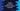# Python symmetric_difference_update example## Python symmetricdifferenceupdate explanation with an example :

symmetricdifferenceupdate method is used to find the symmetric difference of two sets in python.

For two sets setA and setB, the symmetric difference is the set of all elements that are either in setA or setB but not in both.

For example :

``````setA = {1,2,3,4}
setB = {3,4,5,6}``````

The symmetric difference between above two sets is {1,2,5,6}. Only 1,2,5,6 are either in the first set or in the second set.3 and 4 are in both setA and setB.

### Definition :

We can define symmetricdifferenceupdate method as below :

``setA. symmetric_difference_update(setB)``

As you can see that this method takes one Set as the argument. It will return None. It updates the set that is calling, i.e. setA with the result. Or after the method is called, setA will hold the symmetric difference of both sets.

### Example Program :

``````#1
setA = set()
setB = set()

#2
setA_length = int(input(“Enter the size of the first set : “))
setB_length = int(input(“Enter the size of the second set : “))

#3
print(“\n”)
print(“Enter values for the first set one by one : \n”)
for i in range(setA_length):
e = int(input(“Enter value {} : “.format(i+1)))

#4
print(“\n”)
print(“Enter values for the second set one by one : \n”)
for i in range(setB_length):
e = int(input(“Enter value {} : “.format(i+1)))

#5
print(“\nBefore calling symmetric_difference_update : “)
print(“SetA : {} “.format(setA))
print(“SetB : {} “.format(setB))

#6
setA.symmetric_difference_update(setB)

#7
print(“\nAfter symmetric_difference_update is called : “)
print(“SetA : {} “.format(setA))
print(“SetB : {} “.format(setB))``````

### Explanation :

The commented numbers in the above program denote the step numbers below :

1. Create two empty sets setA and setB.
2. Get the length of these sets from the user and put them in setAlength and setBlength.
3. Read the elements of the first set and add them to setA.
4. Similarly, read the elements of the second set and add them to setB.
5. Print the sets to the user.
6. Call symmetricdifferenceupdate to setA and setB using setB as the argument.
7. Print the sets again with the new values in setA.

### Sample Output :

``````Enter the size of the first set : 4
Enter the size of the second set : 4

Enter values for the first set one by one :

Enter value 1 : 1
Enter value 2 : 2
Enter value 3 : 3
Enter value 4 : 4

Enter values for the second set one by one :

Enter value 1 : 3
Enter value 2 : 4
Enter value 3 : 5
Enter value 4 : 6

Before calling symmetric_difference_update :
SetA : {1, 2, 3, 4}
SetB : {3, 4, 5, 6}

After symmetric_difference_update is called :
SetA : {1, 2, 5, 6}
SetB : {3, 4, 5, 6}``````

### Conclusion :

We have learned how to use symmetricdifferenceupdate in python. This method is useful if we need to filter out all common elements from two sets. Try to run the example shown above and drop one comment below if you have any queries.

### Where is the color and why codevscolor ?

Long story short, I love paintings and I paint on weekends. We(me and my wife) have one Youtube channel. Below is a video that I did recently. If you love this please do subscribe to support us 😊